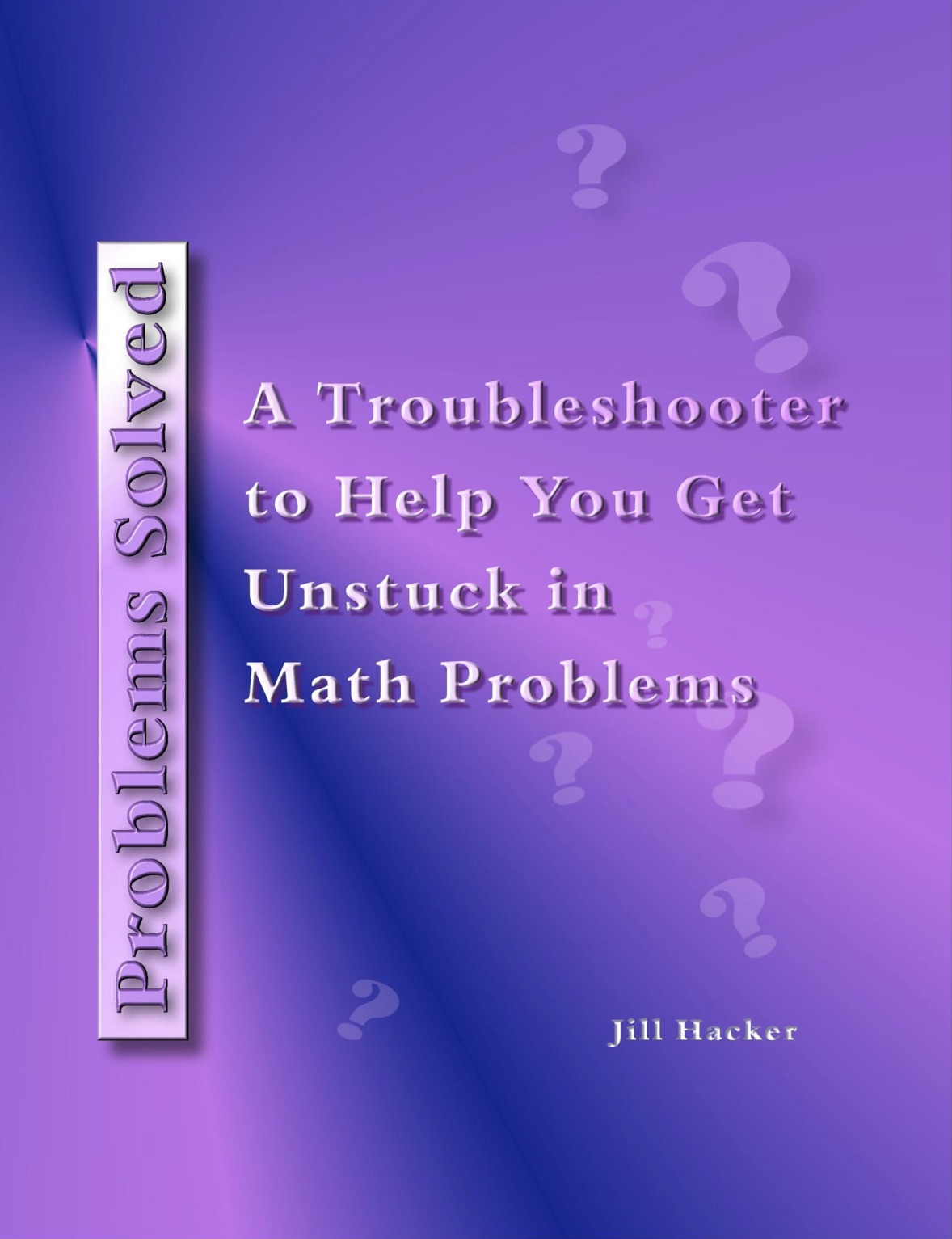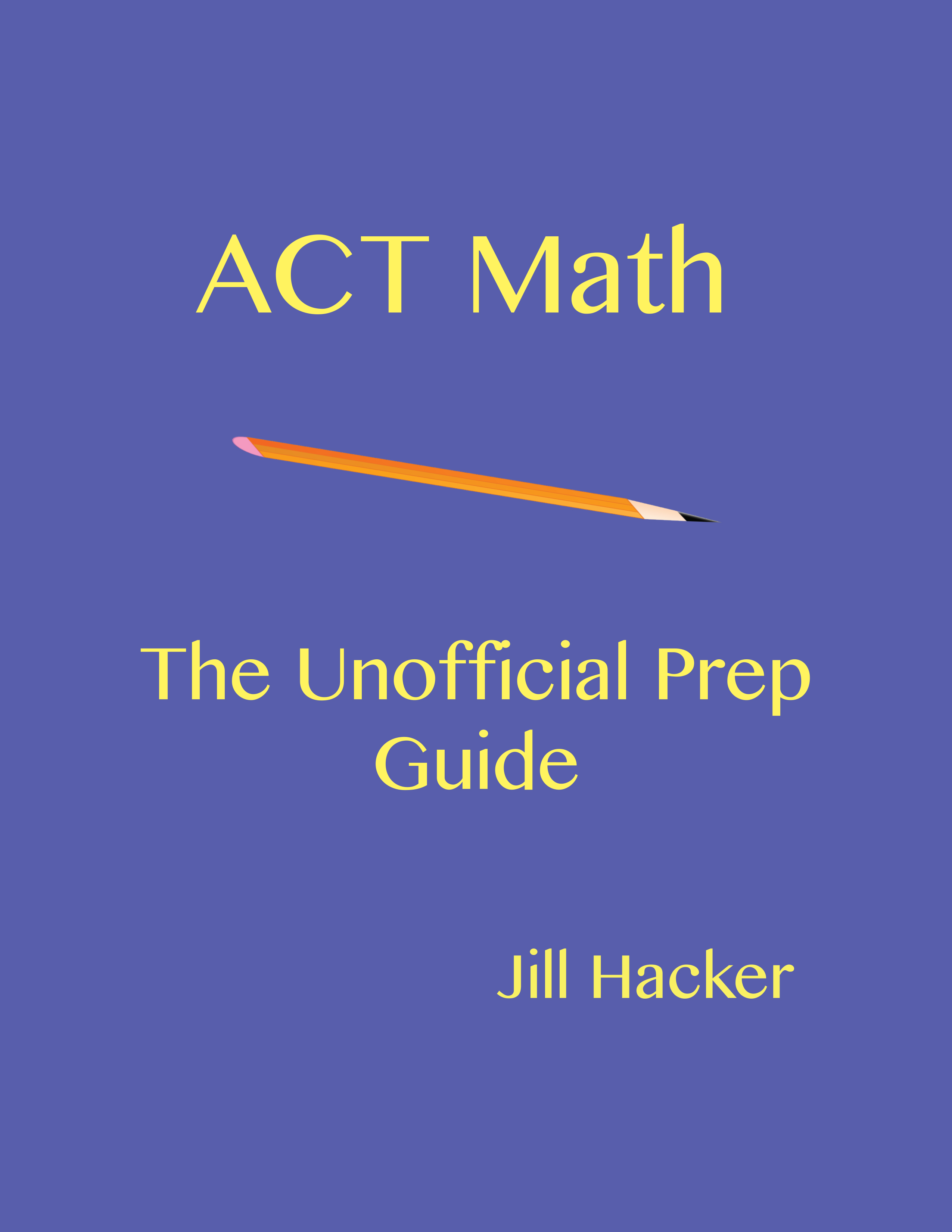## Problems Solved: a Free Math Troubleshooter

Spending too much time stuck in math problems and trying to hunt down your errors? Check out this free math troubleshooter.Problems Solved may be the tool you need. Problems Solved, written for math students from the Algebra 2 level up, is a compilation of common math errors and pitfalls in 22 topic areas. Problems Solved shows you where to look for common small errors, like dropping negative signs and failing to close parentheses on your calculator. Problems Solved explains why: for example, why the change in an exponent’s sign amounts to the same thing as a flip over the fraction bar and why the curve of f(x+1) lies to the left of the curve of f(x). And Problems Solved explains how: for example, how to get radicals and imaginary terms out of denominators and how to handle absolute value in inequalities.

Use Problems Solved as a math troubleshooter and get yourself unstuck in math problems.

Get it FREE.

## ACT Math Unofficial Prep GuideGetting ready for the ACT? Need some support with the math? ACT Math: The Unofficial Prep Guide may be just what you need. ACT Math: The Unofficial Prep Guide contains 60 questions—a whole math section—with a full, reader-friendly explanation for each one. The questions are based on an official ACT practice test, so they cover the full range of ACT topics and difficulty.